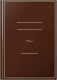# Grade 9 Physics Multiple Choice Questions and Answers (MCQs): Quizzes and Practice Tests with Answer KeyIndependently Published, Mar 11, 2020 - 212 pages

Grade 9 Physics Multiple Choice Questions and Answers (MCQs): Quizzes & Practice Tests with Answer Key (9th Grade Physics Worksheets & Quick Study Guide) covers assessment test worksheets for competitive exam to solve 800 MCQs. "Grade 9 Physics MCQ" with answers covers fundamental concepts with theoretical and analytical assessments tests. "Grade 9 Physics Quiz" PDF, a quick study guide helps to practice test questions for exam review.

"Grade 9 Physics Multiple Choice Questions and Answers (MCQs)" PDF book covers solved quiz questions and answers on topics: Dynamics, gravitation, kinematics, matter properties, physical quantities and measurement, thermal properties of matter, transfer of heat, turning effect of forces, work and energy for school and college level exams. "Grade 9 Physics Questions and Answers" PDF covers exam's workbook, interview questions and certificate exam preparation with answer key.

"9th Grade Physics Worksheets" with answers covers terminology definitions in self-assessment worksheets for physics mock tests on chapters:

Chapter 1: Dynamics MCQs
Chapter 2: Gravitation MCQs
Chapter 3: Kinematics MCQs
Chapter 4: Matter Properties MCQs
Chapter 5: Physical Quantities and Measurement MCQs
Chapter 6: Thermal Properties of Matter MCQs
Chapter 7: Transfer of Heat MCQs
Chapter 8: Turning Effect of Forces MCQs
Chapter 9: Work and Energy MCQs

"Dynamics MCQ" PDF worksheet with answers to solve MCQ practice test: Dynamics and friction, force inertia and momentum, force, inertia and momentum, Newton's laws of motion, friction, types of friction, and uniform circular motion.

"Gravitation MCQ" PDF worksheet with answers to solve MCQ practice test: Gravitational force, artificial satellites, g value and altitude, mass of earth, variation of g with altitude.

"Kinematics MCQ" PDF worksheet with answers to solve MCQ practice test: Analysis of motion, equations of motion, graphical analysis of motion, motion of free falling bodies, rest and motion, scalars and vectors, terms associated with motion, types of motion.

"Matter Properties MCQ" PDF worksheet with answers to solve MCQ practice test: Kinetic molecular model of matter, Archimedes principle, atmospheric pressure, elasticity, Hooke's law, kinetic molecular theory, liquids pressure, matter density, physics laws, density, pressure in liquids, principle of floatation, and what is pressure.

"Physical Quantities and Measurement MCQ" PDF worksheet with answers to solve MCQ practice test: Physical quantities, basic measurement devices, introduction to physics, basic physics, international system of units, least count, significant digits, prefixes, scientific notation, and significant figures.

"Thermal Properties of Matter MCQ" PDF worksheet with answers to solve MCQ practice test: Change of thermal properties of matter, thermal expansion, state, equilibrium, evaporation, latent heat of fusion, latent heat of vaporization, specific heat capacity, temperature and heat, temperature conversion, and thermometer.

"Transfer of Heat MCQ" PDF worksheet with answers to solve MCQ practice test: Heat, heat transfer and radiation, application and consequences of radiation, conduction, convection, and thermal physics.

"Turning Effect of Forces MCQ" PDF worksheet with answers to solve MCQ practice test: Torque or moment of force, addition of forces, angular momentum, center of gravity, center of mass, couple, equilibrium, general physics, principle of moments, resolution of forces, resolution of vectors, torque, and moment of force.

"Work and Energy MCQ" PDF worksheet with answers to solve MCQ practice test: Work and energy, forms of energy, inter-conversion of energy, kinetic energy, sources of energy, potential energy, power, major sources of energy, and efficiency.

### What people are saying -Write a review

We haven't found any reviews in the usual places.